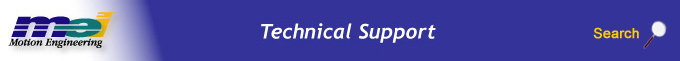.

Post Filter Theory

Laplacian Space

Analog values of the postfilter coefficients are produced as parts of a Laplace Transform:The amplitude and phase of the filter can be derived from the above by: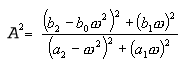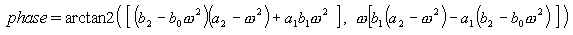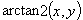is similar to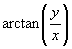except that the returned angle can be in the range from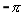to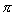.

From here we can calculate the gain (in dB) of the filter: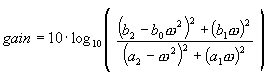The filter types are designed as follows:

 Low Pass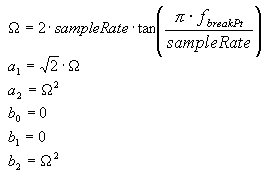High Pass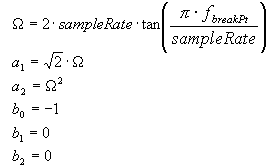Notch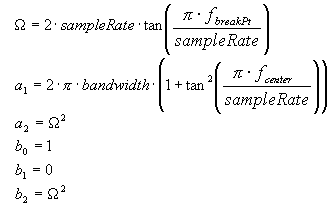Resonator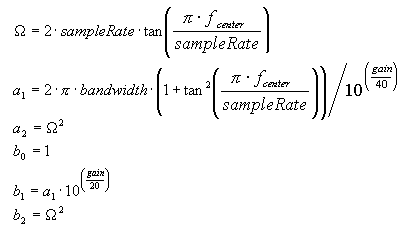Lead, Lag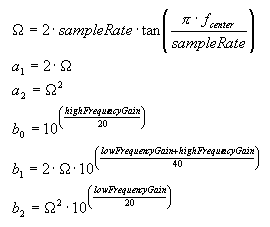For the resonator filter, the maximum and minimum phase changes will occur at: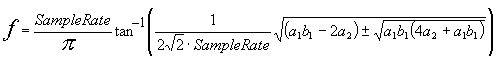These frequencies also happen to be the half-gain points measured in dB (or the root-amplitude gain points).

For the lead/lag filters, the maximal phase change will occur at: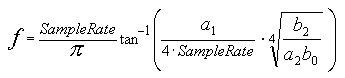This frequency also happens to be the dB gain mean point measured (or the amplitude gain geometric mean point).

Z Space

Though Laplacian Space is useful for designing or quickly analyzing a bi-quad filter's design, it does not accurately model digital bi-quad filters. Digital filters are described naturally by Z transforms. It is possible to convert a filter from a Laplace transform to a Z transform, as will be described below, while maintaining the same general characteristics. The amplitude and phase information will be slightly warped by moving into Z space. One should note, however, that for the filters listed above the characteristics of gains, bandwidths, and center or breakpoint frequencies are unchanged.

Bi-quad filters are described by the following Z transform: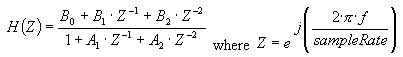One should note that only filters where the roots of the denominator lie within the unit circle are stable. Though digital filters can be constructed where the equations for amplitude and phase for both the Z transform version and the Laplace transform version may converge, the filter itself will be unstable, continually adding energy to the system. Please see the Z Transform Stability Section below.

The equations for amplitude, phase and dB gain can be derived from the above Z transform: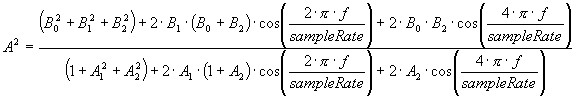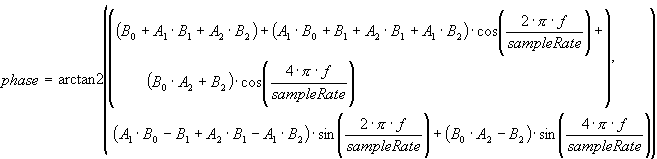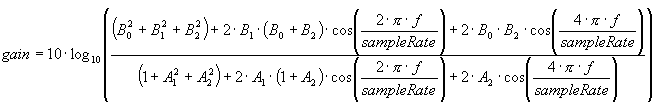The equations for converting between the analog (Laplace transform) coefficients and the digital (Z transform) coefficients are handled internally by the MPI, but are listed below so that one can accurately analyze the performance of the bi-quad filters.

 Bi-quad Postfilter Bi-linear Postfilter (a2 = b2 = 0)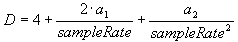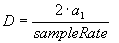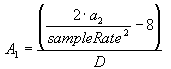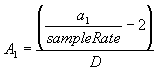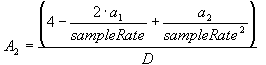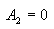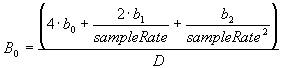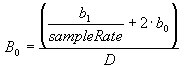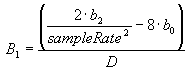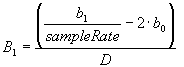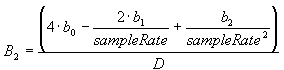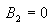Z Transform Stability

As briefly described in the last section, it is possible for the digital filters constructed from analog filters to be unstable. One needs to ensure that:

 The filter does not continually add energy to a system. The filter has no phase lag at 0 frequency. (A filter with 180° phase lag will create unstable closed loop systems.)

To guarantee a filter does not continually add energy to a system, the following relationship must be satisfied by the Z transform coefficients: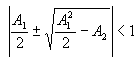To guarantee a filter has no phase lag at 0 frequency, the following relationship must be satisfied by the Z transform coefficients: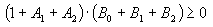If it is found that this last condition is not true, then one should change the sign on all Bn coefficients. Equivalently, one can change the sign of all bn coefficients for the Laplace (analog) transform.